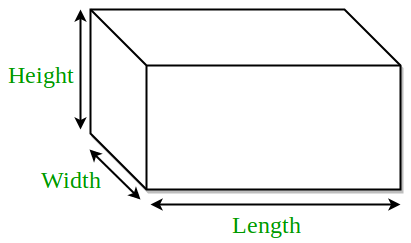# Python Program to find volume, surface area and space diagonal of a cuboid

• Last Updated : 22 Feb, 2022

Given the length, base, and height of a cuboid. The task is to find the Surface Area, Volume and space diagonal of the cuboid.Examples:

```Input :
length = 9
height = 10
Output :
Surface area = 408
volume = 540
space diagonal = 14.73

Input :
length = 5
height = 3
Output :
surface area = 94
volume = 60
space diagonal = 7.07 ```

Formulae Used:

• Surface Area =• Volume =• Space diagonal =Below is the implementation.

## Python3

 `# Python program to find the``# Surface area, volume and``# space diagonal of rectangular``# prism` `import` `math`  `# function to calculate``# Surface area``def` `find_surafce_area(l, b, h):``    ` `    ``# formula of surface_area = 2(lb + bh + hl)``    ``Surface_area ``=` `2` `*` `( l ``*` `b ``+` `b ``*` `h ``+` `h ``*` `l)``    ` `    ``# Display surface area``    ``print``(Surface_area)` `# function to find the``# Volume of rectangular``# prism``def` `find_volume(l, b, h):``    ` `    ``# formula to calculate``    ``# volume = (l * b*h)``    ``Volume ``=` `(l ``*` `b ``*` `h)``    ` `    ``# Display volume``    ``print``(Volume)``    ``categories Most Used`` ``School Programming`` ``Aptitude`` ``Re``def` `find_space_diagonal(l, b, h):``    ` `    ``# formula to calculate``    ``# Space diagonal = square_root(l**2 + b**2 + h**2)``    ``Space_diagonal ``=` `math.sqrt(l``*``*``2` `+` `b``*``*``2` `+` `h``*``*``2``)``    ` `    ``# display space diagonal``    ``print``(Space_diagonal)``    ` `# Driver Code``l ``=` `9``b ``=` `6``h ``=` `10` `# surface area``# function call``find_surafce_area(l, b, h)` `# volume function call``find_volume(l, b, h)``    ` `# Space diagonal function call``find_space_diagonal(l, b, h)``   `

Output:

```408
540
14.730919862656235```

My Personal Notes arrow_drop_up